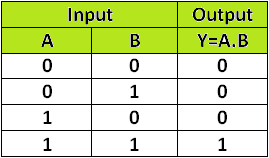# Definition of Logic Gate-:

A logic gate is an idealized or physical device implementing a Boolean function. That it’s performs a basic logical functions and are the fundamental building blocks of digital integrated circuits. Most logic gates take an one or more binary inputs value, and produces a single binary output.

## Noted most important information related to Logic Gates-

1. Supposed it’s two inputs are A and B, which can either be 1 (True) or 0 (False).  In this case the resulting output is a single value of 1 if the result is true, or 0 if the result is false.
2. A logic gate may have more binary input values but its produces a single binary output.
3. At any given moment, every terminal is in one of the two binary conditions low (0) or high (1), represented by different voltage levels.
4. When logic gate is low state condition then value of the logic gate is approximately zero volts (0 V), while the high state is approximately five volts positive (+5 V).

## Primarily implemented & Constructed of Logic Gates are-

•  Diodes or transistors acting as assortment of electronically controlled switches that implement Boolean logic processes.
• Vacuum tubes, electromagnetic relays (relay logic), pneumatic logic, optics, molecules, or even mechanical elements.

## Different Types of Logic Gates### 1. AND Gate

The AND gate is an electronic circuit that gives a High output value (1) only if all its inputs are high. I will be given, two input values from this circuit diagram, that is “A”, “B”  and only one output is “Y”. Here zero (0) is called “False” and one (1) is called “True,  a dot (.) is used to show the AND operation i.e, A.B. The output is “true” when both inputs are “true.” Otherwise, the output is “false.” Total output value express by Y=A.B.

### Truth Table of AND Gate### 2. OR Gate

The OR gate is an electronic circuit or a digital logic gate that gives a high output (1) if one or both the inputs to the gate are high (1). If neither input is high, then output results is low (0)a low. Here zero (0) is called “False” and one (1) is called “True,  a plus (+) is used to show the OR operation i.e, A+B. The output is “False” when both inputs are “False.” Otherwise, the output is “True.” Total output value express by Y=A+B.

### OR Gate with Symbol and Truth Table